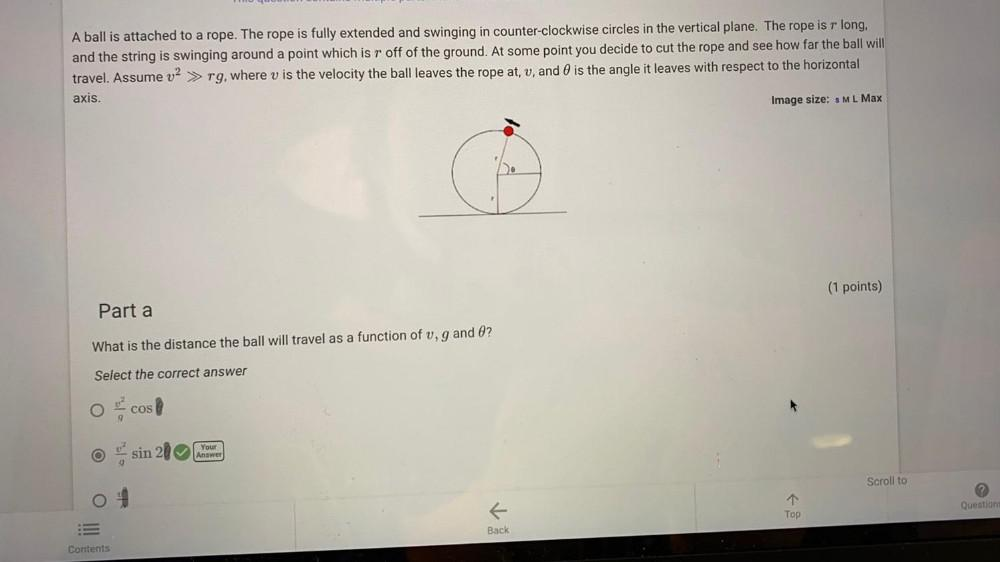Question:

# A ball is attached to a rope. The rope is fully extended and swinging in counter-clockwise circles in the vertical plane. The rope is r long, and the string is swinging around a point which is "r" offA ball is attached to a rope. The rope is fully extended and swinging in counter-clockwise circles in the vertical plane. The rope is r long, and the string is swinging around a point which is "r" off of the ground. At some point you decide to cut the rope and see how far the ball will travel. Assume v²>> rg, where v is the velocity the ball leaves the rope at, v, and θ is the angle it leaves with respect to the horizontal axis. What is the distance the ball will travel as a function of v, g and θ ? (A)v²cosθ/g (B)v² sin2θ/g (C) v²/g